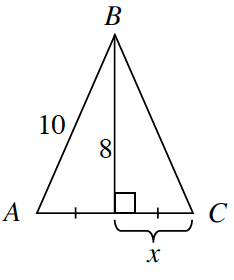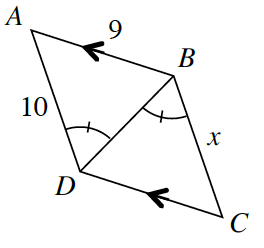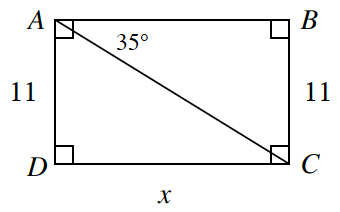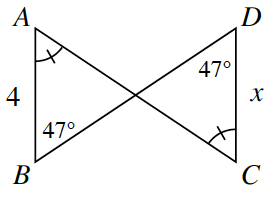### Home > INT2 > Chapter 7 > Lesson 7.1.1 > Problem7-16

7-16.

For each diagram below, determine the value of $x$, if possible. If the triangles are congruent, state which triangle congruence condition you used. If the triangles are not congruent or if there is not enough information, state, “Cannot be determined”.

1. $ABC$ below is a triangle.$ΔABC$ is made of two right triangles with the same base and height. Calculate $x$ using the Pythagorean Theorem.

1.The diagram indicates $\overline{AB}$ and $\overline{DC}$ are parallel. Which angles does that make congruent?

Since the segments are parallel,$∠ABD ≅ ∠BDC$. Therefore, the triangles are congruent by ASA $≅$, so $x = 10$.

1.Since $ΔABC$ is a right triangle, use the tangent ratio to find $AB$. Review the Math Notes boxes in Lessons 3.2.5 and 2.3.2.

1. $\overline{AC}$ and $\overline{BD}$ are straight line
segments.Review the triangle congruence theorems in the Math Notes box in Lesson 2.1.1.